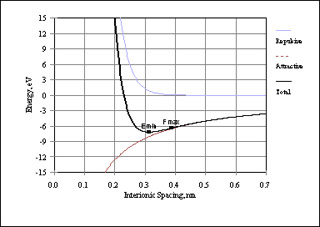# SAMS - Ionic BondingThe NaCl-type structure that is studied in this module.

Abstract: This exercise deals with a classic topic that appears in the first chapters of many introductory materials science text books, ionic bonding in halite (NaCl-type) structures. In addition to illustrating the concepts of bonding in all types of materials, the students show begin to understand the atomic-scale origin of a number of physical properties.

This sample exercise deals only with calculating the energy-distance and lattice force-distance curves and then analyzing them. In the full version the students also get to explore density, coordination numbers, percent ionic bond, and similar concepts that are employed in the energy-distance calculations. These are offered as simpler exercises that lead up to the final modeling exercise.

In this exercise the concepts of attractive and repulsive energies and net lattice energies and forces are introduced and the equations relating lattice energy to selected physical properties are given. The exercise begins by having the students calculate the lattice energy-distance curves and from this the lattice force-distance curves. The results are then analyzed to determine the equilibrium spacing of the atoms and the corresponding lattice energy. In addition, the elastic moduli are calculated and the melting points are estimated. All of these results are then compared to values obtained from the literature. Once satisfactory agreement is had with the case of KCl, the students can then use their spreadsheet to model the behavior of any of the 16 NaCl-type compounds listed in the data tables.

Prerequisite Knowledge: structure of the nature of the atom, coordination numbers, NaCl-type structure, elastic moduli (Y, G, K, and ν), heat capacity.

Spreadsheet Skills: Spreadsheet skills used in this spreadsheet include basic calculations and the use of the natural log and exponential functions (ln and exp). It also involves functions such as min, max and simple numerical techniques such as central difference finite calculus. Basic XY plots are used in these exercises.